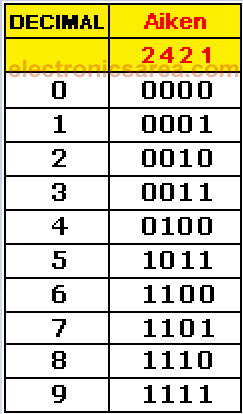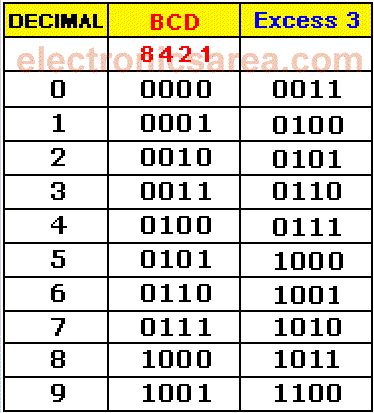# Aiken code

It is recommended to read the tutorial on the Binary number system before you start reading this tutorial. Aiken BCD code is similar to the natural BCD code, but with “weights” or “values” distributed differently. On the natural BCD, weights are: 8 – 4 – 2 – 1. On the Aiken code, the distribution is: 2 – 4 – 2 – 1.

The reason for this coding is to achieve symmetry between certain numbers. View the symmetry in the corresponding Aiken codes for decimal numbers: 4 and 5, 3 and 6, 2 and 7, 1 and 8, 0 and 9.

## Decimal to Aiken Conversion tableEach number is the 9 complement of its symmetrical number, for all digits. The “1” becomes “0” and “0” becomes “1”. For example: 3 (0011) and 6 (1100). We must take into account the new “weights” on this code. The Aiken code is useful for operations of subtraction and division.

You may be interested on: The Gray Code, truth table and applications

# Excess 3 Code

The Excess 3 Code is obtained by adding “3” to each combination of the natural BCD code.

The Excess 3 code is a code where the weighting does not exist (no “weights” as in the natural BCD and Aiken code).

Like the Aiken code, the Excess 3 code  meets the same characteristic of symmetry. Each number is the 9 complement of its symmetrical number, for all digits.

## Decimal to BCD to Excess 3 Conversion tableSee the Excess 3 code symmetry, which corresponds to the decimal numbers: 4 and 5, 3 and 6, 2 and 7, 1 and 8, 0 and 9. It is a useful code in the operations of subtraction and division.

•
•
•
•
•
•
•
•
•
•
•
•
•
•
•
•
•
•